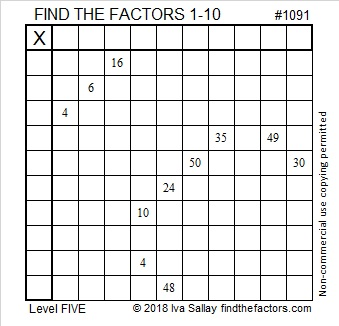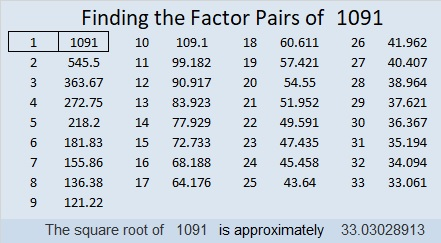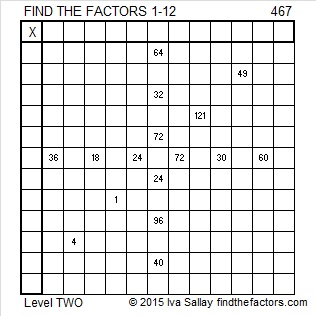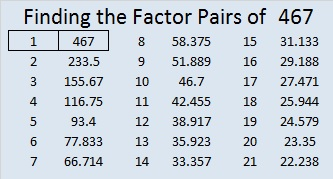# 1091 and Level 5

Can you figure out where to put all the numbers from 1 to 10 in both the first column and the top row so that those factors and the clues can become a multiplication table? Some of the clues might be a little tricky, but I’m sure you can figure them all out.Print the puzzles or type the solution in this excel file: 10-factors-1087-1094

Here are a few facts about the number 1091:

• 1091 is a prime number.
• Prime factorization: 1091 is prime.
• The exponent of prime number 1091 is 1. Adding 1 to that exponent we get (1 + 1) = 2. Therefore 1091 has exactly 2 factors.
• Factors of 1091: 1, 1091
• Factor pairs: 1091 = 1 × 1091
• 1091 has no square factors that allow its square root to be simplified. √1091 ≈ 33.03029

How do we know that 1091 is a prime number? If 1091 were not a prime number, then it would be divisible by at least one prime number less than or equal to √1091 ≈ 33. Since 1091 cannot be divided evenly by 2, 3, 5, 7, 11, 13, 17, 19, 23, 29 or 31, we know that 1091 is a prime number.1091 is the first prime number in the prime triplet (1091, 1093, 1097). It is also the middle number in the prime triplet (1087, 1091, 1093).

1091 looks interesting when it is written in some other bases:
It’s 13331 in BASE 5 because 1(5⁴) + 3(5³) + 3(5²) + 3(5) + 1(1) = 1091,
3D3 in BASE 17 (D is 13 base 10) because 3(17²) + 13(17) + 3(1) = 1091,
and it’s 123 in BASE 32 because 1(32²) + 2(32) + 3(1) = 1091

# What Kind of Prime Is 859?

A prime number is a positive number that has exactly two factors, one and itself. (One has only one factor, so it is not a prime number.)

• 859 is the 149th prime number.

A twin prime is a set of two prime numbers in which the second prime number is two more that the first prime number.

• 859 is the second prime number in the 34th twin prime: (857, 859).

A prime triplet is a set of three consecutive prime numbers in which the last number is six more than the first number. Prime triplets always contain a set of twin primes.

• 859 is in the 27th and 28th prime triplets: (853, 857, 859) and (857, 859, 863).

A prime quadruplet is a set of four consecutive prime numbers in which the last number is eight more than the first number. Prime quadruplets always contain TWO sets of overlapping prime triplets.

• Even though prime numbers (853, 857, 859, 863) contain two sets of overlapping prime triplets, they do NOT form a prime quadruplet because the last number is ten more than the first number. Other than (5, 7, 11, 13), all prime quadruplets are prime decades whose last digits are 1, 3, 7, and 9, in THAT order.

There are other prime constellations like prime quintuplets and prime sextuplets, but each of those has to contain a prime quadruplet in it, so 859 isn’t in any of those.

859÷4 = 214 R3. Since that wasn’t R1, we know that 859 is NOT the hypotenuse of ANY Pythagorean triples.

Now you know what kind of prime 859 is.

Here’s today’s puzzle:Print the puzzles or type the solution on this excel file: 10-factors-853-863

• 859 is a prime number.
• Prime factorization: 859 is prime.
• The exponent of prime number 859 is 1. Adding 1 to that exponent we get (1 + 1) = 2. Therefore 859 has exactly 2 factors.
• Factors of 859: 1, 859
• Factor pairs: 859 = 1 × 859
• 859 has no square factors that allow its square root to be simplified. √859 ≈ 29.3087How do we know that 859 is a prime number? If 859 were not a prime number, then it would be divisible by at least one prime number less than or equal to √859 ≈ 29.3. Since 859 cannot be divided evenly by 2, 3, 5, 7, 11, 13, 17, 19, 23, or 29, we know that 859 is a prime number.

# 467 and Level 2

467 is part of the 22nd prime triplet, (461-463-467). The next prime triplet will not occur until (613, 617, 619).Print the puzzles or type the solution on this excel file:  12 Factors 2015-04-20

—————————————————————————————————

• 467 is a prime number.
• Prime factorization: 467 is prime.
• The exponent of prime number 467 is 1. Adding 1 to that exponent we get (1 + 1) = 2. Therefore 467 has exactly 2 factors.
• Factors of 467: 1, 467
• Factor pairs: 467 = 1 x 467
• 467 has no square factors that allow its square root to be simplified. √467 ≈ 21.61018How do we know that 467 is a prime number? If 467 were not a prime number, then it would be divisible by at least one prime number less than or equal to √467 ≈ 21.6102. Since 467 cannot be divided evenly by 2, 3, 5, 7, 11, 13, 17, or 19, we know that 467 is a prime number.

—————————————————————————————————